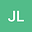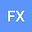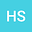Effect of Initial Crack-depth Ratio on Dynamic Fracture Properties of FRP Strengthened Concrete
•••fnq xiangqian
Author Profilehu shaowei
Nanjing Hydraulic Research Institute
Author Profile## Abstract

Abstract: In order to study the dynamic fracture properties of FRP strengthened concrete, the three-point bending experiment of FRP strengthened concrete beams with five different initial crack-depth ratios was carried out by using MTS-810 hydraulic servo experiment machine. The initial crack-depth ratios are 0.2, 0.3, 0.4, 0.5 and 0.6 respectively. The effects of different initial crack-depth ratios on the load-crack mouth opening displacement (CMOD) curves, failure process, increment of critical crack-depth ratio and flexibility coefficient of FRP strengthened concrete beams were analyzed through displacement controlled monotonic loading method. Combined with Pearson correlation experiment formula, the correlation between initial crack-depth ratios and different fracture parameters was quantitatively analyzed. The conclusions could be drawn that, there are three obvious critical points in the fracture damage process of FRP strengthened concrete beams: the starting point of concrete crack, the first peak point and the ultimate bearing capacity point. As the increase of initial crack-depth ratios, the crack initiation load decreases, but the first peak load and ultimate load increase first and then decrease. It is found that the stress intensity factors (SIFs) of the three critical points increase first and then decrease with the increase of the initial crack-depth ratio, and when initial crack-depth ratio of concrete beams is 0.4, three kinds of SIF all reach the maximum value. The increment of critical crack-depth ratio and flexibility coefficient of FRP strengthened concrete beams are related to initial crack-depth ratio, which could be used to reflect the effect of initial crack-depth ratio on the deformation capacity of concrete specimens. Keywords: FRP strengthened concrete; dynamic fracture; initial crack-depth ratios; stress intensity factors; increment of critical crack-depth ratio; flexibility coefficient